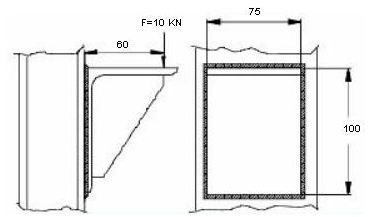# Welding Design Tutorial for Correct Welding Size Calculation and Preparing Welding Drawing for Bending Loads

The procedure for welding size calculation for bending load is a little different than calculation of welding size for pure tension. Here we will discuss the calculation procedure called the "line method" (BS 5950 Clause 6.8.7.2) along with examples.

## A Typical Example

In the picture below one plate is welded over the I-beam and a vertically downward load of 30 KN is acting on the plate.Input Data:

Load F= 10000 N

Length or height of the weld H = 100 mm

Width of the weld B = 75 mm

Design stress of the filler material Fw = 220 N/mm2

Y= H/2 =50 mm

Desired Output:

Throat thickness (t) and leg length (s)

## Steps for Welding Size Calculation by Line Method

• As the load is acting at a distance from the I-beam, the weldment will experience two kinds of stresses, namely bending stress and shear stress.
• Bending moment (M) of the force can be calculated as:

M = 10000 * 60 N-mm

= 600000 N-mm

I = a *(1/6)* (3*B + H )* H2………………Eqn.1

Where,

a is the height of weld section.

For line method height (a ) is assumed to be one and for such case moment of inertia is called unit moment of inertia (Iu), so for line method Eqn.1 become

Unit moment of inertia =Iu = (1/6) * (3*B + H ) * H2………………Eqn.2

• From Eqn.2 calculate the unit moment of inertia (Iu ) of the as:

Iu = 541667.67 mm4

• Calculate bending stress ( Tb) on the welding joint as:

Tb = M * Y / Iu

= 55.384 N/ mm2

• Calculate unit area of the welding (Au) as:

Au = (B+H)*2

= 350 mm2

• Calculate shear stress ( Ts) on the welding joint as:

Ts = F/Au

= 28.571 N/ mm2

• Calculate resultant (T) of bending stress and shear stress on welding as:

T = (Tb2+Ts2) 1/2

=62. 32 N/ mm2

• Next, calculate throat thickness (t) as:

t = T/Fw

= 0.283 mm

• Finally, calculate welding leg length (s) using the following equation:

s = 1.414 * t

= 0.4 mm (approximately 0.5 mm)

## Conclusion

Welding design calculation procedure explained in the welding design tutorial can be used for any bending load problem provided you have calculated the applied force correctly. Alternatively or for validating your design, FEA tools can also be used.

## Related Reading

• AWS Welding Symbols for Five Most Useful Weld Types: AWS welding symbols are widely accepted for welding drawing. AWS symbols and ANSI symbols are same. All the AWS weld symbols are derived from the standard called AWS A2.4. We will discuss about five important weld joints types and their AWS welding symbols in this article.